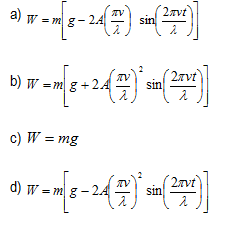# Friction Multiple Choice questions

## Paragraph Based Questions

(A) A particle of weight W moves under the action of a force
F = A cos ωt
where A and ω are constant at x = 0 and v = 0 at t = 0
Question 1
The velocity time relation is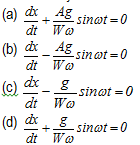Question 2
The displacement time equation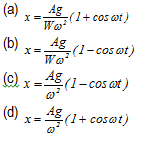## Matrix Match type question

Question 3
A pendulum of length l and mass m is supported from the ceiling of the elevator. Let T0 be the time period of oscillation when the elevator is still
Column A
(a) elevator is moving up with constant velocity
(b) elevator is moving down with constant velocity
(c) elevator is moving up with constant acceleration
(d) elevator is moving down with constant acceleration
Column B
(P) T = T0
(Q) T > T0
(R) T < T0
(S) no appropriate match

Question 4
Let
S1 = frame of reference at rest
S2 = frame of reference at constant velocity
S3 = frame of reference at constant acceleration
S4 = frame of reference at uniform circular motion
A block A is at rest as seen from frame of reference S1
Column A
(a) S1
(b) S2
(c) S3
(d) S4
Column B
(P) ΣF ≠ 0
(Q) ΣF =0
(R) a = 0
(S) a ≠ 0
where ΣF is the resultant force and a is the acceleration of the body

Question 5
Consider the figures given below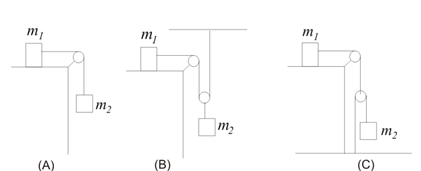Column A
(a) Acceleration of mass m2 in (A)
(b) Acceleration of mass m1 in (B)
(c) Acceleration of mass m2 in (C)
(d) Acceleration of mass m2 in (B)
Column B
(P) (m2g)/(4m1 + m2)
(Q) (m2g)/(m1 + m2)
(R) (2m2g)/(4m1 + m2)
(S) (m2g)/(2m1 + m2)

## Paragraph Based questions

(B) A,B, C are the objects as shown above in the figure. A, B, C are 2, 3 and 4 Kg respectively. Coefficient of friction between the blocks are given above in the figure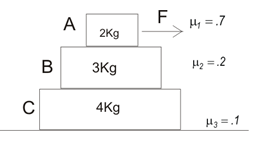Let $f_1$ be frictional forces between A & B
$f_2$ be frictional force between B & C
$f_3$ be frictional force between C & surface
Let $a_1$, $a_2$, $a_3$ be the acceleration of A ,B  and C respectively
Question 6
Which one of the following is true
(a) 0≤ f1 ≤  14, 0≤ f2  ≤ 10, 0≤ f3  ≤ 9
(b) 0≤ f1  ≤ 14, 0≤ f2  ≤ 6, 0≤ f3  ≤ 4
(c) 0≤ f1  ≤ 14, 0≤ f2  ≤ 4, 0≤ f3  ≤ 6
(d) 0≤ f1  ≤ 14, 0≤ f2  ≤ 10, 0≤ f3  ≤ 6

Question 7
What is the minimum force F to have so that all part moves with non zero acceleration
(a) 9N
(b) 10N
(c) 14N
(d) 11N

Question 8
If F = 12 N, which of the following is true
(a) $f_1 = 11.2N$, $f_2 = 10N$, $f_3 = 9N$
$a_1= a_2 = a_3 = 0.4 \ m/s^2$
(b) $f_1 = 11.2N$, $f_2= 10N$, $f_3 = 9N$
$a_1 = a_2 = 0.4 \ m/s^2$, $a_3 = 0.25 \ m/s^2$
(c) $f_1 = 11N$, $f_2 = 10N$, $f_3 = 0$
$a_1 = a_2 = a_3= 0.45 \ m/s^2$
(d) none of the above

Question 9
what is the minimum force required to have relative motion between B & C object
(a) 11.5
(b) 10
(c) 11.25
(d) none of the above

Question 10
what is the minimum force required to have relative motion between A & B object
(a) 17.5
(b) 16.6
(c) 14
(d) none of the above

Question 11
for F = 15 N
(a) there will be relative motion between A & B
(b) there will be relative motion between B & C
(c) there will be relative motion between C & surface
(d) none of the above

(C)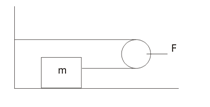The pulley is assumed as mass less and friction free.
Question 12
Find the acceleration of the block assuming no friction is present
(a) F/2m
(b) F/m
(c) 2F/m
(d) none of the above

Question 13
If friction force F/4 exist between the block and surface
(a) F/m
(b) F/m
(c) F/4m
(d) none of the above

(D) A particle of charge Q and mass M with an initial velocity v0i enter an electric field
E=-Ej
Question 14
What force act in x and y direction
(a) Fx = 0, Fy = -QE j
(b) Fx = 0, Fy = QE j
(c) Fx = qE i, Fy = 0
(d) Fx = -qE i, Fy = 0

Question 15
Velocity at time t is given by
(a) (v0 + qE/M)i
(b) v0 i + (qEt/M) j
(c) v0i – (qEt/M) j
(d) none of the above

Question 16
Let us assume that particle is at origin at t = 0, find the position vector at t = at time t
(a) v0t i – (qEt2/2M) j
(b) v0t i + (qEt2/2M) j
(c) (v0t + qEt2/2M)i
(d) none of the above

(E)A block of Mass M rests on smooth horizontal surface over which it can move without friction. A body of mass m lies on the block. The coefficient of friction between body and block is K.  The force F acts in horizontal direction on the block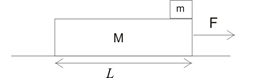Question 17
For what values of F, both the bodies will move together without any relative motion
(a) 0 ≤  F  ≤ kMg
(b) 0 ≤  F  ≤ kmg
(c) 0 ≤  F  ≤ k(M+m)g
(d) None of these

Question 18
When the Force F is sufficient to have relative motion between the block and body, what will the acceleration of block and body?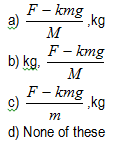Question 19
Find the time in which body will fall from the block when relative motion is present. Assume L is the length of the block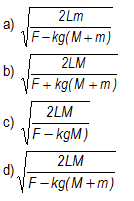## Multiple Choice Questions

Question 20
A spring balance is attached to the roof of the car and a mass m is hanging from it. When the car is standing on the horizontal road, the balance correctly tells us the weight of the mass. The car is travelling with a constant horizontal velocity v on the undulating path defined by the function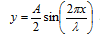where y is the height above road surface.
Find the weight of the mass in spring balance as function of time t. We can assume that while going up on the undulating surface, the car floor remains almost horizontal